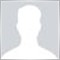# Help with Bitwise shift left MQL4 book example13

 char a='a',b='b';   Print("Before:  a = ",a, "  b = ",b); //--- shift to the left   b=a<<1;   Print("After:   a = ",a, "  b = ",b); // The result will be:// Before:  a = 97   b = 98// After:   a = 97   b = -62

I get that the output of variable b is going to be the binary value of a (which is 97 and therefore 01100001 in binary form) shifted to the left by 1.

Now this is where I get stuck, the output of -62 gives 11000001 in binary form, which is not the 011000010 I was expecting

Could someone explain the reasoning behind this, thank you in advance38661

A char is between 127 and -128. The first bit (on the left) is used to sign the value (0 is +, 1 is -).

97 shifted by 1 to the left is 11000010.

So -10000000 + 1000010 : -128 + 66 = -62102

Mike Dean:

...the output of -62 gives 11000001 in binary form, which is not the 011000010 I was expecting...

What Alain forgot to mention is that you experience the cutoff of left bits which do not fit into the type char's limited range (-127...128). Your leftmost zero gets lost because a char holds only 8 bits. Whenever you shift to left/right everything that crosses the boundaries of your actual type get's lost. Imagine it as if there is not "enough place" in your type.